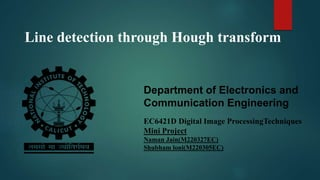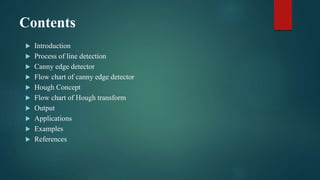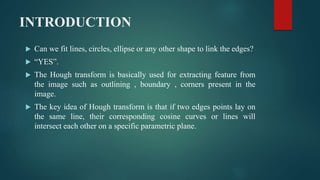Seu SlideShare está sendo baixado. ×

# Line Detection using Hough transform .pptx

Anúncio
Anúncio
Anúncio
Anúncio
Anúncio
Anúncio
Anúncio
Anúncio
Anúncio
Anúncio
AnúncioCarregando em…3
×

1 de 30 Anúncio

# Line Detection using Hough transform .pptx

This ppt describes the line detection using the concept of Hough Transform in Digital image Processing

This ppt describes the line detection using the concept of Hough Transform in Digital image Processing

Anúncio
Anúncio

### Line Detection using Hough transform .pptx

1. 1. Department of Electronics and Communication Engineering EC6421D Digital Image ProcessingTechniques Mini Project Naman Jain(M220327EC) Shubham loni(M220305EC) Line detection through Hough transform
2. 2. Contents  Introduction  Process of line detection  Canny edge detector  Flow chart of canny edge detector  Hough Concept  Flow chart of Hough transform  Output  Applications  Examples  References
3. 3. INTRODUCTION  Can we fit lines, circles, ellipse or any other shape to link the edges?  “YES”.  The Hough transform is basically used for extracting feature from the image such as outlining , boundary , corners present in the image.  The key idea of Hough transform is that if two edges points lay on the same line, their corresponding cosine curves or lines will intersect each other on a specific parametric plane.
4. 4. Input image Edge detection Hough transform Output Image Process of Line Detection
5. 5. Canny Edge Detector  The Canny edge detector is an edge detection method that has multistage algorithm to detect the wide range of edges in an image  It was developed by John F. Canny in 1986.  Canny edge detection is a technique to extract useful structural information from different vision objects and dramatically reduce the amount of data to be processed.  It has been widely applied in various computer visionsystems.
6. 6. Flow chart of canny edge detector • Using Gaussian operator Noise Reduction • Using Sobel operator Edge detection Non – maximum suppression Double Thresholding Hysteresis Edge tracking
7. 7. Noise Reduction :  It is basically a smoothing technique.  In an image noise consist of sharp transition in intensity  To eliminate those noise spatial filters are used such as box filter , weighted averages and Gaussian filter.  Gaussian smoothing is widely used.
8. 8. Edge detection:  The Gradient operator is used . Mag(img) Phase (img)
9. 9. Non-maximum suppression:  Original phase is Quantize into one of the four different phases.  Go in the direction specified by the gradient ,then check the neighbouring pixel .  If lower then the centre pixel then treated as edge.
10. 10. Double Thresholding and Hysteresis:  Here we are taking Double Thresholding instead of standard way of threshold.  The double threshold step aims at identifying 3 kind of pixels :strong ,weak and non relevant .  Strong:- pixels having intensities so high that they are surely contribute to final edge.  Weak:- pixels having intensities so low , that is not enough to be consider as strong ones but yet not small enough to be consider non- relevant to be an edge.  Other pixels are consider to be non-relevant to the edge.
11. 11. Canny Edge Image
12. 12. Hough Transform • Elegant method for direct object recognition • Edges need not be connected • Complete object need not be visible • Key Idea: Edges VOTE for the possible model
13. 13. Flow chart of Hough transform: Input Edge image Mapping edge points to parametric space Creating ACUMULATOR Find the point with maximum voting Detection of Line
14. 14. Hough Transform: Concept
15. 15. Line Grouping Problem
16. 16. The Straight Line (x1,y1) (x2,y2) • For each point (x , y) in the line the following equation applies: • Therefore: 𝑦 = 𝑚 ⋅ 𝑥 + 𝑐
17. 17. Multiple Lines over a single point  Each pair (m , b) defines a distinct straight line containing the point (x,y) (x,y) y=m1x+b1 y=m2x+b2 y=m3x+b3 y=m4x+b4
18. 18. The Parameter Plane  Each point in the (x,y) space(image plane) is mapped to a straight line in the (m ,b) space (parameter plane).  A straight line in the (x,y) space (image plane) is mapped to the intersection point of the lines corresponding to its points, in the (m.b) space (parameter plane). b =-mx1+y1 (x1,y1) (x2,y2) (x3,y3) b =-mx3+y3 b =-mx2+y2
19. 19. The Accumulator Concept  The (m.b) space (parameter plane) is subdivided in cells.  Each pixel (x,y) in the original image vote in the (m, b) space for each line passing through it.  The votes are summed in an Accumulator m mmin mmax b1 m1 N Corresponds to a straight line y=m1x+b1 of N pixels length b
20. 20. The Motivation for Polar Coordinates  Vertical lines cannot be mapped to the (m,b) space, since:  Vertical lines can be described using polar coordinates : x = r r ?    b m xcos0 + ysin0 = r
21. 21. Using Polar Coordinates • For each point (x,y) in the line the following equation applies: • In particular: (x1,y1) (x2,y2) r θ       sin cos sin cos sin cos 2 2 1 1 y x r y x r y x r         sin cos y x r  
22. 22. r = x · cos θ + y · sin θ For given point (0,2) , (2,0), (1,1)
23. 23. Input Image Output Image Output
24. 24. Examples
25. 25. Applications  It is widely used in feature extraction (shapes of the objects).  Another big advantage is used for the building-edge extraction.  It can extract the lines even through the noisy data.  By the Hough transform, we can detect the missing lines which are broken while detecting edge image.  We can use it in lane detection of the road.
26. 26. REFRENCE  Names: Gonzalez, Rafael C., author. | Woods, Richard E. (Richard Eugene), author  Wikipedia, Internet search  https://ieeexplore.ieee.org/document/6949962 DOI :- 10.1109/ICCSP.2014.6949962  https://ieeexplore.ieee.org/document/9422200 DOI :- 10.1109/TPAMI.2021.3077129
27. 27. Thank You